# NCERT Solutions for Class 12 Mathemetics Chapter 1 - Relations and Functions

##### Question 1:

Determine whether each of the following relations are reflexive, symmetric and transitive : Relation R in the set A = {1, 2, 3, ......, 13, 14} defined as : R = {(x, y) : 3x – y = 0}

Here
A = {1, 2, 3, ....... , 13, 14}
and R = {(x, y) : 3x – y = 0}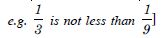##### Question 2:

Determine whether each of the following relations are reflexive, symmetric and transitive :
Relation R in the set N of natural numbers defined as :
R = {(x, y) : y = x + 5 and x < 4}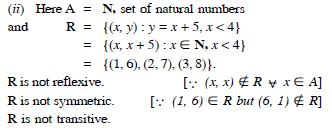##### Question 3:

Determine whether each of the following relations are reflexive, symmetric and transitive :
Relation R in the set A = {1, 2, 3, 4, 5, 6} defined as :
R = {(x, y) : y is divisible by x }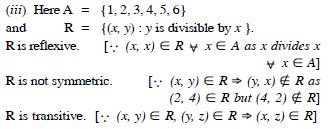##### Question 4:

Determine whether each of the following relations are reflexive, symmetric and transitive :
Relation R in the set Z of all integers defined as :
R = {(x, y) : x – y is an integer}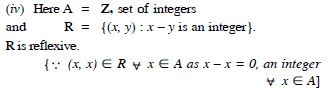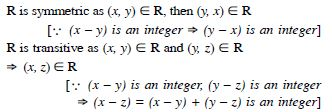##### Question 5:

Determine whether each of the following relations are reflexive, symmetric and transitive :
Relation R in the set A of human beings in a town at a particular time given by :
(a) R = {(x, y) : x and y work at the same place}
(b) R = {(x, y) : x and y live in the same locality}
(c) R = {(x, y) : x is exactly 7 cm taller than y}
(d) R = {(x, y) : x is wife of y}
(e) R = {(x, y) : x is father of y}.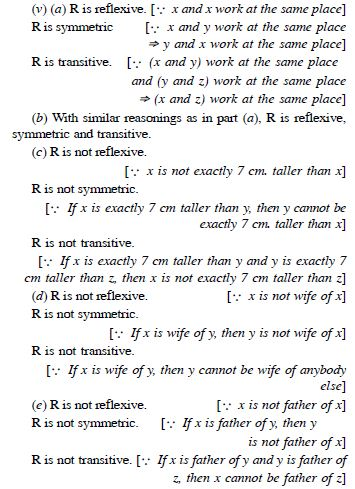##### Question 6:

Show that the relation R in the set R of real numbers, defined as :
R = {(a, b) : a $\le$ b2} is neither reflexive nor symmetric nor transitive.

R is not reflexive.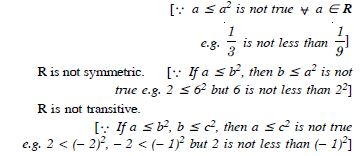##### Question 7:

Check whether the relation R defined in the set {1, 2, 3, 4, 5, 6} as :
R = {(a, b) : b = a + 1}, is reflexive, symmetric or transitive.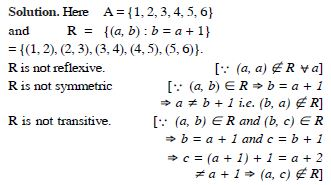##### Question 8:

Show that the relation R in R defined as :
R = {(a, b) : a $\le$ b}
is reflexive and transitive but not symmetric.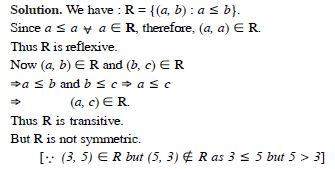##### Question 9:

Check whether the relation R in R defined by :
R = {(a, b) : a $\le$ b3}
is reflexive, symmetric or transitive.

R is not reflexive.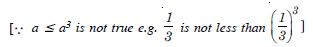R is not symmetric.
[$\therefore$ If a $\le$ b3, then b is not less than or equal to a3
e.g. 1 $\le$ 33. But 3 is not less then 13]
R is not transitive.
[$\therefore$ If a $\le$ b3 and b $\le$ c3, then a is not necessarily less than or equal to c3
e.g. Take a = 7, b = 2, c = 1·5.
Here a < b3 as 7 < 23 = 8
b < c3 as 2 < (1·5)3 = 3.375
But a > c3 as 7 > (1·5)3 = 3·375]

##### Question 10:

Show that the relation R in the set {1, 2, 3} defined as :
R = {(1, 2), (2, 1)}, is symmetric but neither reflexive nor transitive.

Here R = {(1, 2), (2, 1)}.
R is not reflexive. [$\therefore$ (1, 1), (2, 2), (3, 3) $\notin$ R]
R is not transitive.
[$\therefore$ (1, 2) $\in$ R, (2, 1) $\in$ R but (1, 1) $\notin$ R]
R is symmetric. [$\therefore$ (1, 2) $\in$ R and (2, 1) $\in$ R]

##### Question 11:

Show that the relation R in the set A of all books in a library of a college, given by :
R = {(x, y) : x and y have same number of pages} is an equivalence relation.

We have :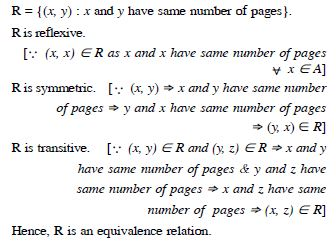##### Question 12:

Show that the relation in the set A = {1, 2, 3, 4, 5}, given by :
R = {(a, b) : | a – b | is even} is an equivalence relation.
Show that all the elements of {1, 3, 5} are related to each other and all the elements of {2, 4} are related to each other. But no element of {1, 3, 5} is related to any element of {2, 4}.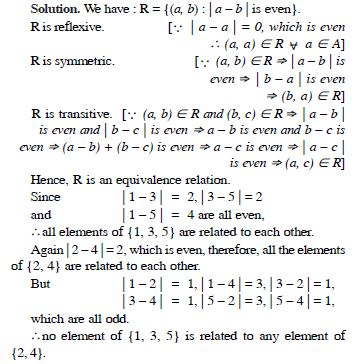##### Question 13:

Show that each of the relation R in the set :
A = {x : x $\in$ Z, 0 $\le$ x $\le$ 12}, given by :
(i) R = {(a, b) : | a – b | is a multiple of 4}
(ii) R = {(a, b) : a = b}
is an equivalence relation.
Find the set of all elements related to 1 in each case.

Here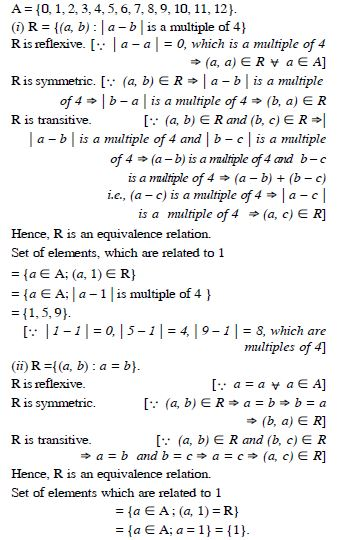##### Question 14:

Give an example of a relation, which is :
Symmetric but neither reflexive nor transitive

Let A = {1, 2, 3}.
The relation R = {(2, 3), (3, 2)} is symmetric but neither reflexive nor transitive.
[$\therefore$ (1, 1) $\notin$ R; (2, 3), (3, 2) $\in$ R but (2, 2) $\notin$ R]

##### Question 15:

Give an example of a relation, which is :
Transitive but neither reflexive nor symmetric

Let A = {1, 2, 3}.
The relation R = {(1, 3), (3, 2), (1, 2)} is transitive but neither reflexive nor symmetric.
[$\therefore$ (1, 1) $\notin$ R; (1, 3) $\in$ R but (3, 1) $\notin$ R]

##### Question 16:

Give an example of a relation, which is :
Reflexive and symmetric but not transitive

Let A = {1, 2, 3}.
The relation R = {(1, 1), (2, 2), (3, 3), (2, 3), (3, 2), (1, 2), (2, 1)}
is reflexive and symmetric but not transitive.
[$\therefore$ (1, 2) and (2, 3) $\in$ R but (1, 3) $\notin$ R]

##### Question 17:

Give an example of a relation, which is :
Reflexive and transitive but not symmetric

Let A = {1, 2, 3}.
The relation R = {(1, 1), (2, 2), (3, 3), (1, 2)} is reflexive and transitive but not symmetric.
[$\therefore$ (1, 2) $\in$ R but (2, 1) $\notin$ R]

##### Question 18:

Give an example of a relation, which is :
Symmetric and transitive but not reflexive

Let A = {1, 2, 3}.
The relation R = {(1, 2), (2, 1), (1, 1), (2, 2)} is symmetric and transitive but not reflexive. [$\therefore$ (3, 3) $\notin$ R]

##### Question 19:

Show that the relation R in the set A of points in a plane, given by :
R = {(P, Q) : distance of the point P from the origin is same as the distance of the point Q from the origin} is an equivalence relation.
Further, show that the set of all points related to a point P $\ne$ (0, 0) is the circle passing through A with origin as centre.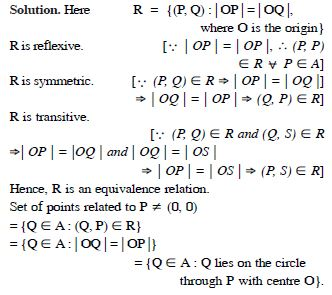##### Question 20:

Show that the relation R, defined by the set A of all triangles as :
R = {(T1, T2) : T1 is similar to T2] is an equivalence relation.
Consider three right angle triangles T1 with sides 3, 4, 5; T2 with sides 5, 12, 13 and T3 with sides 6, 8, 10.
Which triangles among T1, T2 and T3 are related?

Here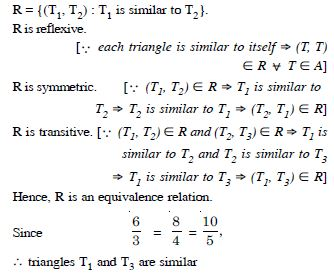$⇒$ T1 is related to T3 and T3 is related to T1
$⇒$ (T1, T3) $\in$ R.

##### Question 21:

Show that the relation related to R, defined in the set of all polygons as :
R = {(P1, P2), P1 and P2 have same number of sides} is an equivalence relation.
What is the set of all elements in A related to the right triangle T with sides 3, 4 and 5 ?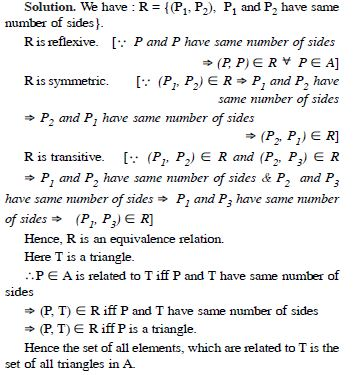##### Question 22:

Let L be the set of all lines in XY-plane and R is the relation in L defined as :
R = {(L1, L2) : L1 is parallel to L2}.
Show that R is an equivalence relation.
Find the set of all lines related to the line y=2x + 4.

We have :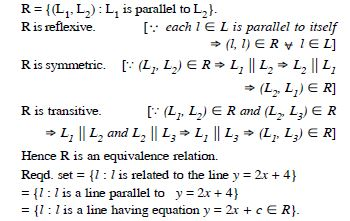##### Question 23:

Let R be the relation in the set {1, 2, 3, 4} given
by : R = {(1, 2), (2, 2), (1, 1), (4, 4), (1, 3), (3, 3), (3, 2)}.

1. R is reflexive and symmetric but not transitive
2. R is reflexive and transitive but not symmetric
3. R is symmetric and transitive but not reflexive
4. R is an equivalence relation

R is reflexive and transitive but not symmetric

##### Question 24:

Let R be the relation in the set N given by :
R = {(a, b) : a = b – 2, b > 6}.

1. (2, 4) $\in$ R
2. (3, 8) $\in$ R
3. (6, 8) $\in$ R
4. (8, 7) $\in$ R.

(6, 8) $\in$ R

##### Question 25:

Show that the function f : R* $\to$ R* defined by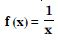is one-one and onto, where R* is the set of all nonzero real numbers. Is the result true if the domain R* is replaced by N with co-domain having same as R* ?

Let x1, x2 $\in$ R*.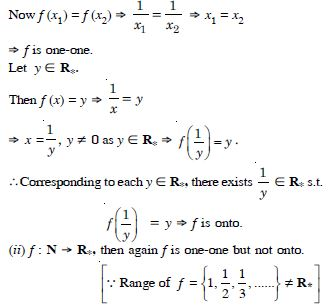##### Question 26:

Check the injectivity and surjectivity of the following function :
f : N $\to$ N given by f (x) = x2

Let x1, x2 $\in$ N.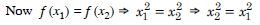$⇒$ − + (x2 x1 ) (x2 x1 ) = 0 $⇒$ x2 – x1 = 0
[$\therefore$ x1, x2 $\in$ N $\therefore$ x1 + x2 $\ne$ 0]
$⇒$ x1 = x2 $⇒$ f is one-one
$⇒$ f is injective.
Now range of f = {12, 22, 32, ...}
= {1, 4, 9, ...} $\ne$ N
$⇒$ f is not onto $⇒$ f is not surjective.

##### Question 27:

Check the injectivity and surjectivity of the following function :
f : Z $\to$ Z given by f (x) = x2

Let x1, x2 $\in$ Z.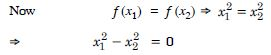$⇒$ x1 = x2 or x1 = – x2
$⇒$ x2 = x1 or x2 = – x1.
Thus f (x1) = f (– x1) −∨
x1 $\in$ Z
$⇒$ f is not one-one $⇒$ f is not injective.
Also range of f = {0, 12, 22, ...} = {0, 1, 4, ...} $\ne$ Z
$⇒$ f is not onto $⇒$ f is not surjective.

##### Question 28:

Check the injectivity and surjectivity of the following function :
f : R $\to$ R given by f (x) = x2

Let x1, x2 $\in$ R.
As in part (ii), f is not injective.
Also range of f = {0, 12, 22, ...}
= {0, 1, 4, ...} $\ne$ R
$⇒$ f is not onto $⇒$ f is not surjective.

##### Question 29:

Check the injectivity and surjectivity of the following function :
f : N $\to$ N given by f (x) = x3

Let x1, x2 $\in$ N.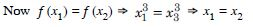$⇒$ f is one-one $⇒$ f is injective.
Now range of f = {13, 23, 33, ...} = {1, 8, 27, ...} $\ne$ N
$⇒$ f is not onto $⇒$ f is not surjective.

##### Question 30:

Check the injectivity and surjectivity of the following function :
f : Z $\to$ Z given by f (x) = x3

Let x1, x2 $\in$ Z.
As in part (iv), f is injective.
Now range of f = {13, 23, 33, ...} = {1, 8, 27, ...} $\ne$ Z
$⇒$ f is not onto $⇒$ f is not surjective.

##### Question 31:

Show that the Greatest Integer Function f : R $\to$ R given by :
f (x) = [x], is neither one-one nor onto, where [x] denotes the greatest integer less than or equal to x.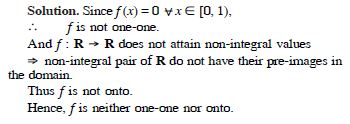##### Question 32:

Show that the Modulus Function f : R $\to$ R, given by :
f (x) = | x |
is neither one-one nor onto, where | x | is x, if x is positive and | x | is – x, if x is negative.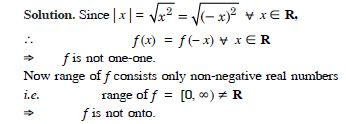##### Question 33:

Show that the Signum function f : R $\to$ R given by :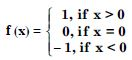is neither one-one nor onto.

Since f (x) =1 for all x $\in$ (0, $\infty$)
and f (x) = – 1 for all x $\in$ (– $\infty$, 0),
$\therefore$ f is many-one $⇒$ f is not one-one.
Also range of f = {– 1, 0, 1) $\ne$ R
$⇒$ f is not onto.

##### Question 34:

Let A = {1, 2, 3}, B = {4, 5, 6, 7} and let f = {(1, 4),
(2, 5), (3, 6)} be a function from A to B. Show that f is oneone.

We have : f = {(1, 4), (2, 5), (3, 6)}.
Then f (1) = 4, f (2) = 5 and f (3) = 6
$⇒$ Different points of domain correspond to different f-images in the range.
Hence, f is one-one.

##### Question 35:

In each of the following cases, state whether the function is one-one, onto or bijective. Justify your answer.
(i) f : R $\to$ R defined by f (x) = 3 – 4x
(ii) f : R $\to$ R defined by f (x) = 1 + x2.

(i) Let x1, x2 $\in$ R.
Now f (x1) = f (x2)
$⇒$ 3 – 4x1 = 3 – 4x2
$⇒$ x1 = x2 $⇒$ f is one-one.
Let y $\in$ R. Let y = f (x0).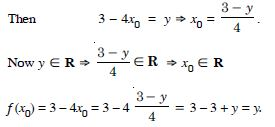$\therefore$ For each y $\in$ R, there exists x0 $\in$ R such that f (x0) = y.
$\therefore$ f is onto.
Hence, ‘f’ is one-one and onto or bijective.
(ii) Here f (1) = 1 + 1 = 2,
f (– 1) = 1 + 1 = 2.
Now 1 $\ne$ – 1 but f (1) = f (– 1)
$\therefore$ f is not one-one.
Also range of f is [1, $\infty$) $\ne$ R
$\therefore$ f is not onto.
Hence, ‘f’ is not bijective.

##### Question 36:

Let A and B be sets. Show that :
f : A × B $\to$ B × A such that f (a, b) = (b, a) is a bijective function.

(a1, b1), (a2, b2) $\in$ A × B such that
f (a1 , b1 ) = (a2, b2 )
$⇒$ (b1 , a1 ) = (b2, a2 )
$⇒$ b1 = b2 and = a1 = a2
$⇒$ (a2, b2 ) = (a1 , b1 )
$\therefore$ f is one-one.
And corresponding to each ordered pair (y, x) $\in$ B × A,
there exists (x, y) $\in$ (A × B) such that
f (x, y) = (y, x)
$\therefore$ f is onto.
Hence, ‘f ’is a bijective function.

##### Question 37:

Let f : N $\to$ N be defined by :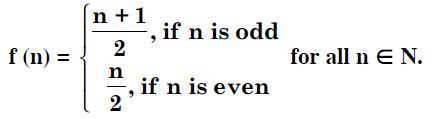State whether the function f is onto, one-one or bijective. Justify your answer.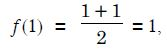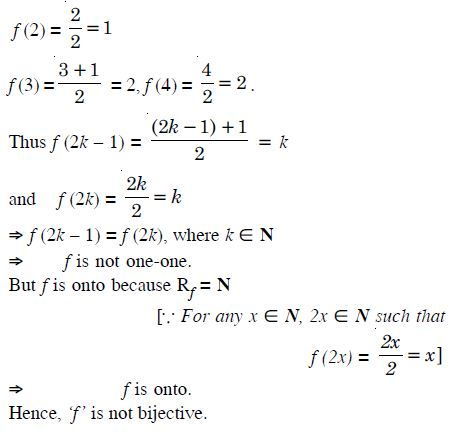##### Question 38:

Let A = R – {3} and B = R – {1}.
Consider the function f : A $\to$ B defined by :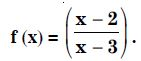Let x1, x2 $\in$ R – {3}.
Now 1 f (x ) = f (x2 )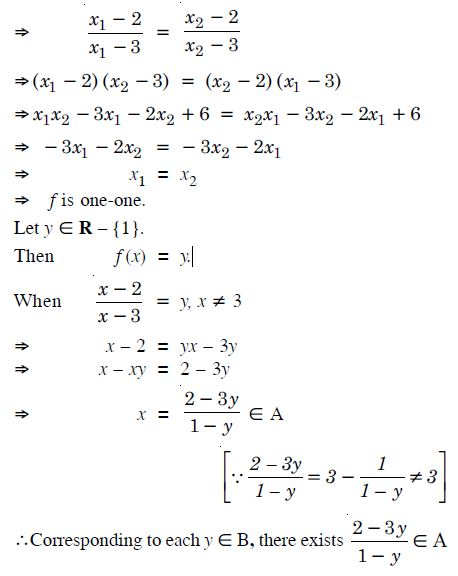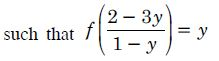$⇒$ f is onto.
Hence, ‘f’ is one-one and onto.

##### Question 39:

Let f : R $\to$ R be defined as f (x) = x4.

1. f is one-one onto
2. f is many-one onto
3. f is one-one but not onto
4. f is neither one-one nor onto.

f is neither one-one nor onto.

##### Question 40:

Let f : R $\to$ R be defined as f (x) = 3x.

1. f is one-one onto
2. f is many one onto
3. f is one-one but not onto
4. f is neither one-one nor onto.

f is one-one onto

##### Question 41:

Let f : {1, 3, 4} $\to$ {1, 2, 5} and g : {1, 2, 5} $\to$ {1, 3}
be given by :
f = {(1, 2), (3, 5), (4, 1)
and g = {(1, 3), (2, 3), (5, 1)}.
Write down gof.

We have : f : {1, 3, 4} $\to$ {1, 2, 5}
and g : {1, 2, 5} $\to$ {1, 3}.
Here f (1) = 2, f (3) = 5 and f (4) = 1
and g (1) = 3, g (2) = 3 and g (5) = 1.
Here Rf = {1, 2, 5} = Dg
$\therefore$ Dgof = Df = {1, 3, 4}.
Now (gof) (1) = g (f (1)) = g (2) = 3
(gof) (3) = g (f (3)) = g (5) = 1
and (gof) (4) = g (f (4)) = g (1) = 3.
Hence, (gof) : {(1, 3), (3, 1), (4, 3)}.

##### Question 42:

Let f, g and h be functions from R to R. Show that :
(i) (f + g) oh = foh + goh
(ii) (f.g) oh = (foh) . (goh).

(i) For all x $\in$ R,
[( f + g) oh] (x) = ( f + g) (h(x))
= f (h(x))+g(h(x))= ( foh) (x)+(goh)(x)
=[( foh)+(goh)] (x).
Hence, ( f + g) oh = foh+ goh.
(ii) For all x $\in$ R,
(( f .g) oh) x = ( f .g) (h(x))
= f (h(x)) g (h(x))
= ( foh) (x) . (goh) (x)
= [( foh) . (goh)] (x).
Hence ( f .g) oh = ( foh) . (goh) .

##### Question 43:

Find gof and fog, if :
(i) f (x) = | x | and g (x) = | 5x – 2 |
(ii) f (x) = 8x3 and g (x) = x1/3.

(i) (a) gof (x)= g ( f (x))
= g (|x|) = |5|x|−2|.
(b) fog (x) = f (g (x)) = f (|5x −2|)
= |5x −2|.
(ii) (a) gof (x) = g ( f (x)) = g(8x3 )
=(8x3)1/3 =2x .
(b) fog (x) = f (g (x))
f(x1/3) = 8(x1/3)3 =8x

##### Question 44: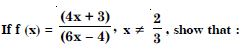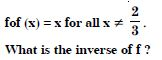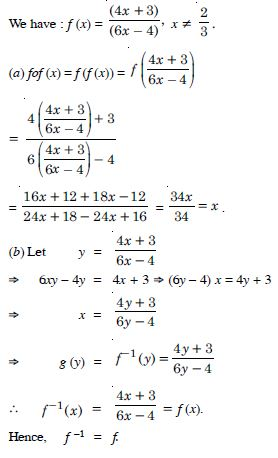##### Question 45:

State with reasons whether following functions have inverse :
(i) f : {1, 2, 3, 4} $\to$ {10} with
f = {(1, 10), (2, 10), (3, 10), (4, 10)}
(ii) g : {5, 6, 7, 8} $\to$ {1, 2, 3, 4} with
g = {(5, 4), (6, 3), (7, 4), (8, 2)}
(iii) h : {2, 3, 4, 5} $\to$ {7, 9, 11, 13} with
h = {(2, 7), (3, 9), (4, 11), (5, 13)}.

(i) Here f (1) = f (2) = f (3) = f (4) = 10,
$\therefore$ f is not one-one
[$\therefore$1, 2, 3, 4 have same image 10]
$⇒$ f is many-one
$⇒$ f has no inverse.
(ii) Here f (5) = f (7) = 4
$\therefore$ f is not one-one [$\therefore$5 and 7 have same image 4]
$⇒$ f is many-one
$⇒$ f has no inverse.
(iii) Here each element of {2, 3, 4, 5} has a unique element in {7, 9, 11, 13}.
Similarly each element of {7, 9, 11, 13} has a unique preimage in {2, 3, 4, 5}.
$⇒$h is one-one onto $⇒$ f is invertible.
Hence, ‘h’ has the inverse.

##### Question 46:

Show that : f [– 1, 1] $\to$ R given by :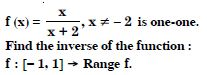f (x1) = f (x2 )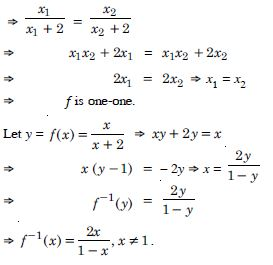##### Question 47:

Consider f : R $\to$ R given by f (x) = 4x + 3. Show that f is invertible.
Find the inverse of f.

We have : f (x) = 4x + 3.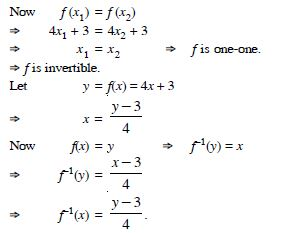##### Question 48:

Let f : R $\to$ R be defined by f (x) = 3x – 7. Show that f is invertible.
Find f –1 : R $\to$ R.

We have : f (x) = 3x – 7.
Now f (x1) = f (x2 )
$⇒$ 3x1 – 7 = 3x2 – 7
$⇒$ x1 = x2 $⇒$ f is one-one
$⇒$ f is invertible.
Let y = f (x) = 3x – 7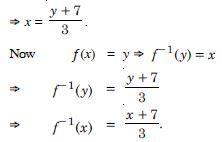##### Question 49:

Consider R $\to$ [4, $\infty$) given by f (x) = x2 + 4. Show that f is invertible with the inverse –1 f of f given by f –1 (y) =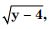where R* is the set of all nonnegative

real numbers.

f (x1)= f (x2)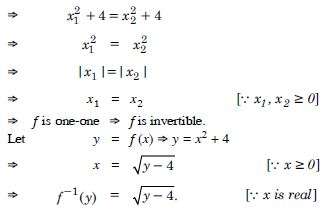##### Question 50:

Consider f : R $\to$ [– 5, $\infty$), given by :
f (x) = 9x2 + 6x – 5.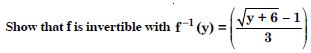f (x1) = f (x2 )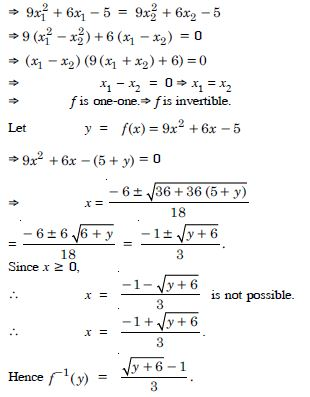##### Question 51:

Let f : X $\to$ Y be an invertible function. Show that f has unique inverse.

Since f is invertible, [Given]
$\therefore$ f is one-one onto.
If ‘g’ be the inverse of ‘f’, then
gof (x) = IX and fog (y) = IY.
Let ‘g1’, and ‘g2’ be two inverses of ‘f’.
$\therefore$ fog1 (y) = IY and fog2 (y) = IY
$⇒$ g1 (y) = g2 (y)
[Q f is one-one onto]
Hence ‘f’ has a unique inverse.

##### Question 52:

Consider f : {1, 2, 3} $\to$ {a, b, c}, given by :
f (1) = a, f (2) = b and f (3) = c.
Find f –1 and show that (f –1 )–1 = f.

We have : f (1) = a, f (2) = b, f (3)= c.
Thus f = {(1, a), (2, b), (3, c)}.
Clearly, f is one-one $⇒$ f is invertible
and 1 = f−1 (a), 2 = f−1 (b),
3 = f−1 (c)
$⇒$ f−1 : {a, b, c} $\to$ {1, 2, 3}.
Clearly, f−1 is one-one and onto
and ( f−1)−1 ={(1, a), (2, b), (3, c)}
[$\therefore$ f−1 = {(a, 1), (b, 2), (c, 3)}]
$⇒$ ( f −1)−1 = f.

##### Question 53:

Let f : X $\to$ Y be an invertible function. Show that the inverse of f–1 is f i.e. (f–1 )–1 = f.

f : X $\to$ Y is invertible
$⇒$ f is one-one and onto.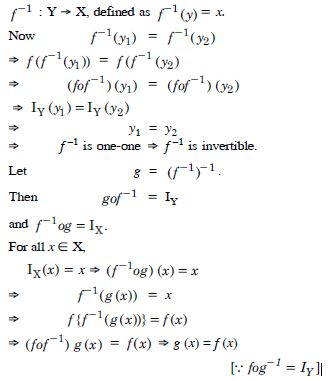$⇒$ g = x.
Hence ( f−1)−1 = f.

##### Question 54:

If f : R $\to$ R be given by f (x) = (3 – x3 )1/3 , then f of (x) is :

1. x1/3
2. x3
3. x
4. (3 – x3 )

x

##### Question 55: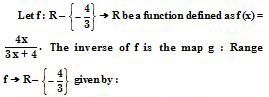1.2.3.4.##### Question 56:

Let R be the relation in the set {1, 2, 3, 4}, given by :
R = {(1, 2), (2, 2), (1, 1), (4, 4), (1, 3), (3, 3), (3, 2)}.
Then :

1. R is reflexive and symmetric but not transitive
2. R is reflexive and transitive but not symmetric
3. R is symmetric and transitive but not reflexive
4. R is an equivalence relation.

R is reflexive and transitive but not symmetric

##### Question 57:

Let R be the relation in the set N given by :
R = {(a, b) : a = b – 2, b > 6}.
Then :

1. (2, 4) $\in$ R
2. (3, 8) $\in$ R
3. (6, 8) $\in$ R
4. (8, 7) $\in$ R.

(6, 8) $\in$ R

##### Question 58:

Let A = {1, 2, 3}. Then number of relations containing {1, 2} and {1, 3}, which are reflexive and symmetric but not transitive is :

1. 1
2. 2
3. 3
4. 4

1

##### Question 59:

Let A = {1, 2, 3}. Then the number of equivalence relations containing (1, 2) is :

1. 1
2. 2
3. 3
4. 4

2

##### Question 60:

Let f : R $\to$ R be defined as f (x) = x4. Then :

1. f is one–one onto
2. f is many–one onto
3. f is one–one but not onto
4. f is neither one–one nor onto.

f is neither one–one nor onto.

##### Question 61:

Let f : R $\to$ R be defined as f (x) = 3x. Then :

1. f is one–one onto
2. f is many–one onto
3. f is one–one but not onto
4. f is neither one–one nor onto.

f is one–one onto

##### Question 62:

If f : R $\to$ R be given by f (x) = (3 − x31/3, then fof (x) is :

1. x1/3
2. x3
3. x
4. 3 – x3

x

##### Question 63: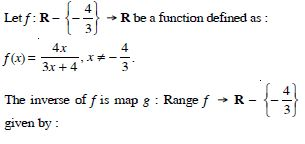1.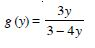2.3.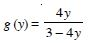4.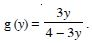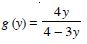##### Question 64:

Consider a binary operation ‘*’ on N defined as :
a * b = a3 + b3. Then :

1. is ‘*’ both associative and commutative ?
2. is ‘*’ commutative but not associative ?
3. is ‘*’ associative but not commutative ?
4. Is ‘*’ neither commutative nor associative ?

is ‘*’ commutative but not associative ?

##### Question 65:

Number of binary operations on the set {a, b} is :

1. 10
2. 16
3. 20
4. 8